## Drilling Fluids Calculations For Drilling & Mud Engineer|| Oil Field Consulting Services Houston | Alberson Cement Consulting

On: September 23, 2022

# Drilling Fluids Calculations For Drilling & Mud Engineer

In this article we introduce a simple guide for drilling fluid calculations for mud engineering, preparations, system, volumes and more.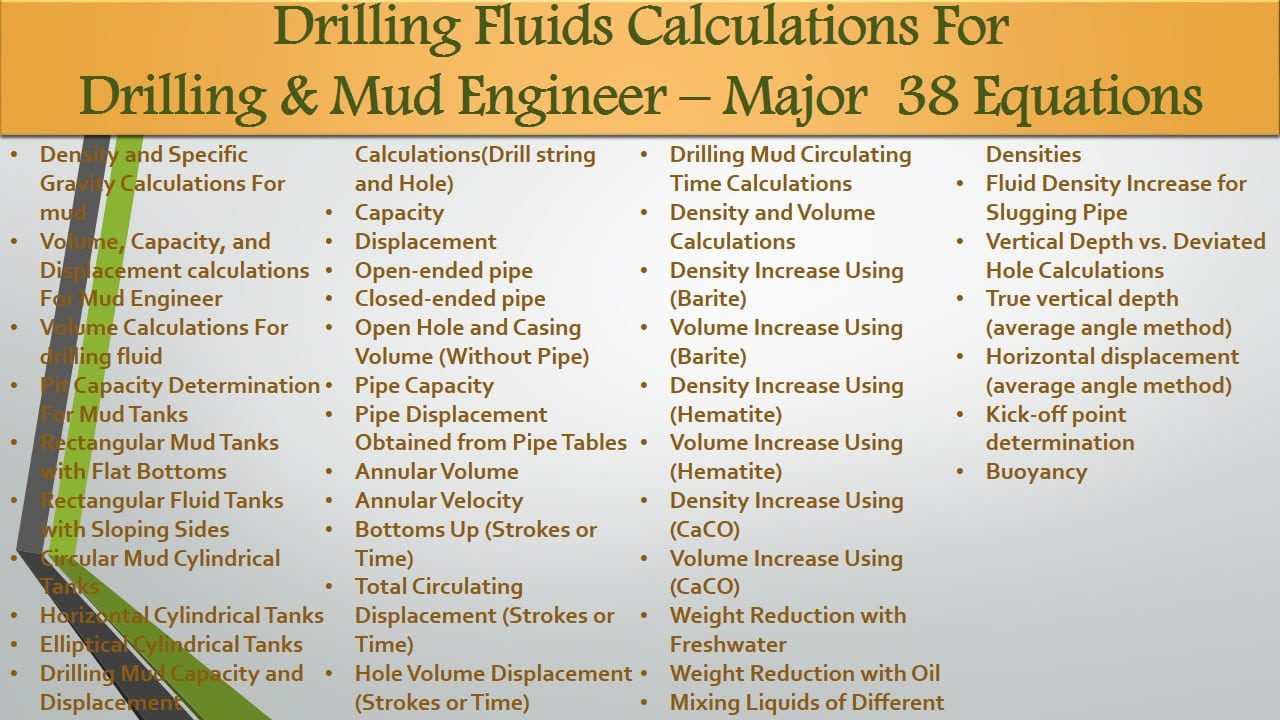Mud Engineers & drilling engineers need to have quick drilling fluid calculations equations to help them while they are on the rig. In this article, we will have a simple

guide for the most used equations for drilling mud preparations, system, displacement, volumes, and more.

## Basic Drilling Fluid / Mud Engineering Calculations

### Density and Specific Gravity Calculations For mud

The density of a material is defined as its mass per unit volume and is usually expressed in units of g/cm3, kg/m3 or, commonly in oilfield usage for liquids, lb/US gal. As an example, the density of (barite) is 4 g/cm3. Specific gravity (SG) is a unitless measure of relative density (the density of a material divided by the density of a reference substance).

The reference substance is almost always water which has a density very close to 1 g/cm3 and so the specific gravity of a material is very similar numerically to the density of that material but for accurate work, it is necessary to quote the temperatures at which measurements are made.

To determine the SG of a material, divide the density of the material by the density of water. Note that for accurate work, the density of water is dependent on temperature and the reference temperature should be quoted. Common reference temperatures are 39°F (4°C) and 68°F (20°C); the difference in the density of water at these two temperatures is approximately 0%.

Two figures are widely used in the drilling fluids industry for the density of water in oilfield units (lb/US gal). The figure of 45 assumes that the temperature of measurement is 39°F (4°C) and this is the figure adopted by the API. The figure of 3 assumes that the temperature of measurement is 68°F (20°C) and this is the figure used throughout this article. Using this figure, a 15.0 lb/gal mud has an SG of 1.8.

Equation 1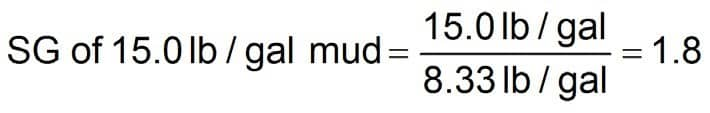### Volume, Capacity, and Displacement calculations For Mud Engineer

Volume and capacity have the same units, but are not always equal. For example, a mud pit of 300 bbl capacity empty might only contain a volume of 200 bbl. Displacement is the volume of fluid displaced when the drill string is run into the wellbore full of fluid.

#### Volume Calculations For drilling fluid

Mud system volume = the total volume of what is in the active pits plus that volume in the hole

Total mud volume = pit volume + hole volume

Pump output volume = that volume adjusted for pump efficiency. Duplex mud pumps have an approximate efficiency of 85–95%, whereas triplex pumps have an approximate efficiency of 90–98%.

The following two drilling equations which is used by the mud engineer calculate pump output Q, at 100% volumetric efficiency. The constant, Ve, can be adjusted to calculate the bbl/stroke, gal/stroke, or liter/stroke.

Equation 2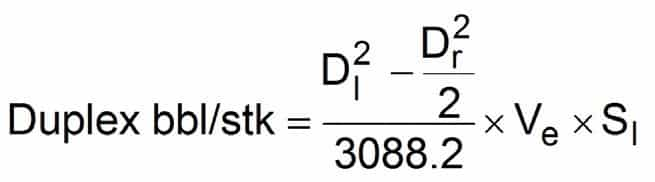Equation 3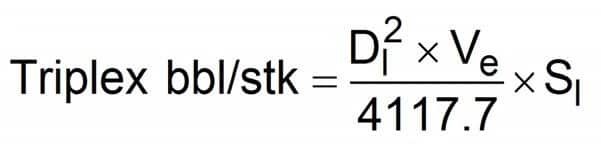Where:

Dl = Liner diameter, inches

Dr = Rod diameter, inches

Ve = Volumetric efficiency (decimal fraction)

Sl = Stroke length, inches

### Pit Capacity Determination For Mud Tanks

The most common container for the surface volumes is rectangular pits with vertical sides and flat bottoms. Other types of pits that may be encountered are rectangular pits with sloping sides and circular or elliptical cylindrical tanks. ( see also the drilling fluid system article)

All dimensions used by the drilling/mud engineers in the following calculations formulae are in feet.

#### Rectangular Mud Tanks with Flat Bottoms

Equation 4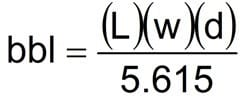Drilling Fluids Calculations For Rectangular Mud Tanks

#### Rectangular Fluid Tanks with Sloping Sides

Equation 5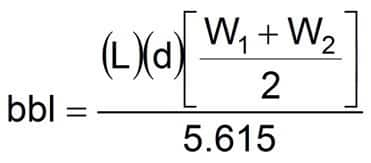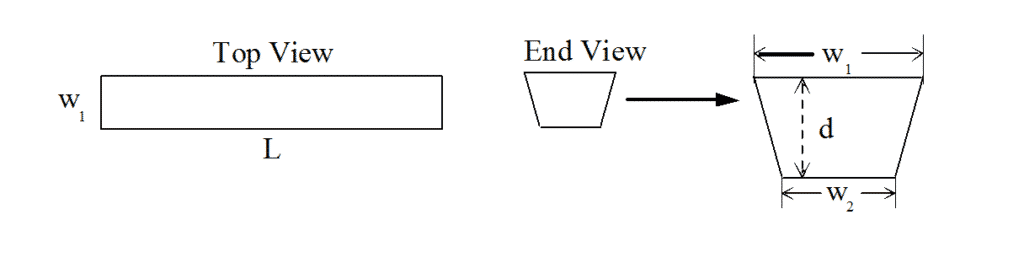#### Circular Mud Cylindrical Tanks

For mud engineer calculations, Upright Cylindrical Tanks

Equation 6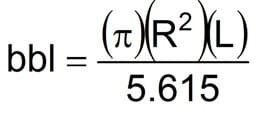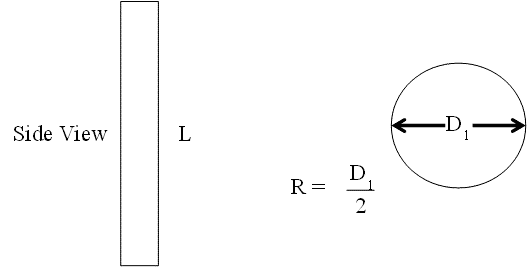Drilling Fluids Calculations For Circular Mud Cylindrical Tanks

#### Horizontal Cylindrical Tanks

i. Determine the total tank capacity.

Equation 7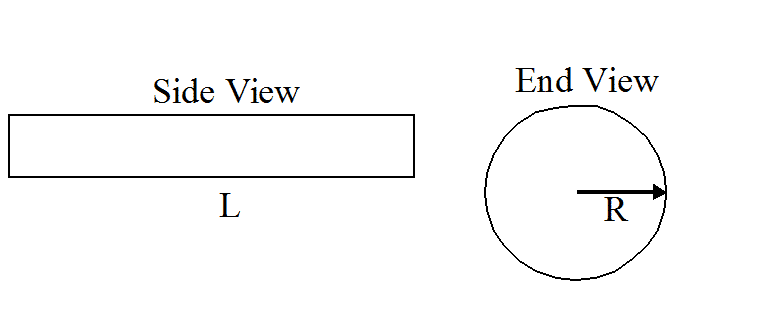ii. Determine the percentage of tank diameter that is filled. If an 8 ft diameter tank is filled to a 2 ft level, the tank is filled to 25% of its diameter.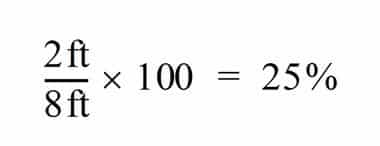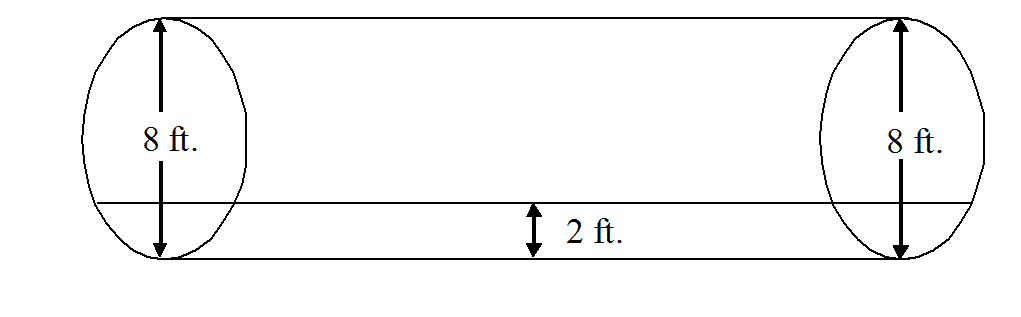iii. Use the following graph to determine the tank volume based on percentage of tank diameter filled.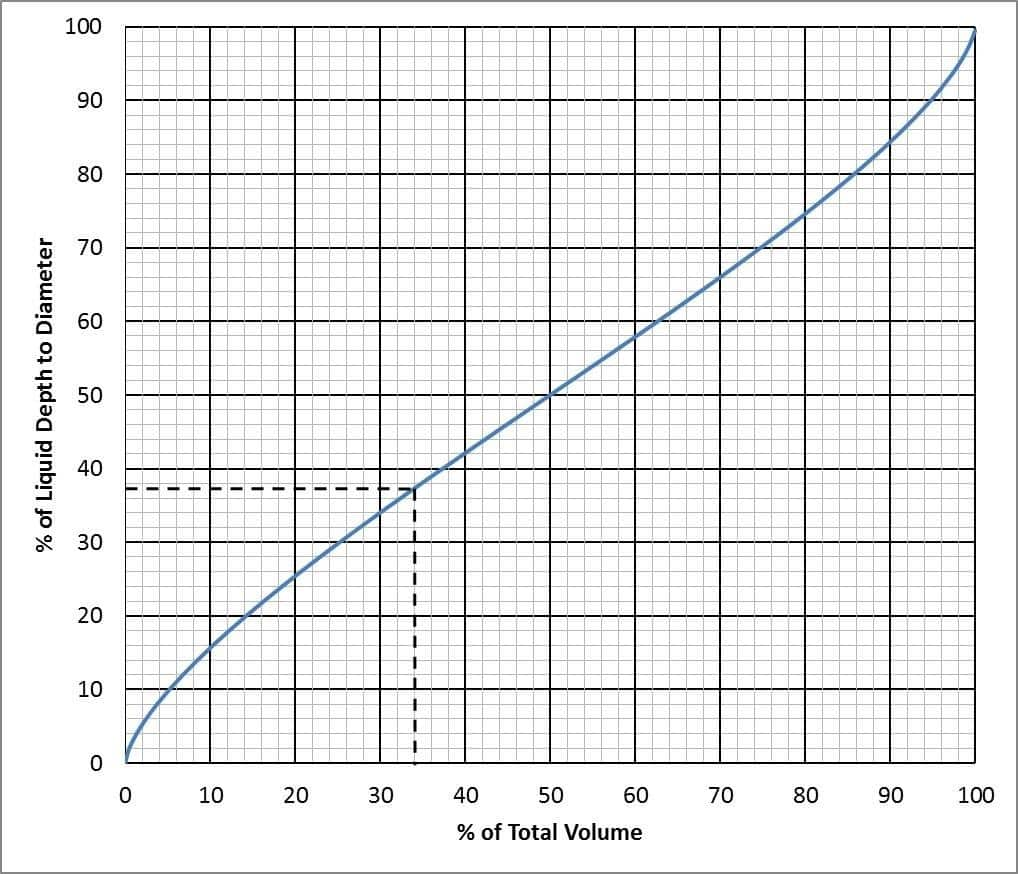Figure 1: Drilling Fluids Calculations Calibration for Cylindrical Tanks in a Horizontal Position

Example:

Diameter = 4 ft

Length = 20 ft

Fluid height = 1 ft

Total tank capacity = (34 × 22 × 20)/515 = 443 bbl

Percentage of liquid depth to diameter = (1 ÷ 4) × 100 = 37

From the graph, a percentage of 37 indicates 34% of capacity

Percentage of total capacity = 04×44 = 15 bbl fluid in tank

#### Elliptical Cylindrical Tanks

Equation 8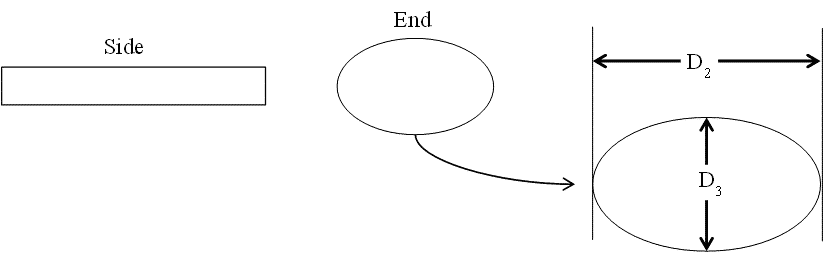Drilling Fluids Calculations For Elliptical Cylindrical Tanks

## Drilling Mud Capacity and Displacement Calculations(Drill string and Hole)

### Nomenclature

• Outside diameter, in. — OD
• Inside diameter, in. — ID
• Hole diameter, in. — Dh
• Annular outer pipe diameter, in. — D
• Annular inner pipe diameter, in. — d
• Closed-ended pipe — CE
• Open-ended pipe — OE

### Capacity

For drilling/mud Engineer to do calculations of the capacity of drill pipe, drill collars, Heavy weight drill pipe, Casing, other tubular, wellbore (cased or open) (Download Now Capacity Calculator Sheet): Diameter of wellbore = Dh or casing ID are used to solve the following equation.

Equation 9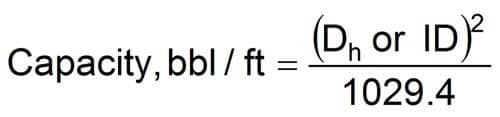### Displacement

#### Open-ended pipe

As related to drill pipe, drill collars, and other tubulars is the volume of fluid that the pipe displaces by its wall thickness. Pipe OD and ID are used in the following equation:

Equation 10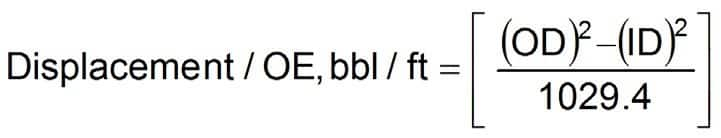#### Closed-ended pipe

As related to drill pipe, drill collars and other tubular is the volume of fluid that is displaced by its wall thickness plus the internal capacity of the pipe.

Equation 11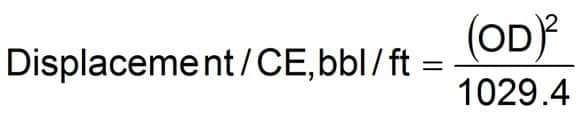### Open Hole and Casing Volume (Without Pipe)

Equation 12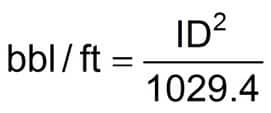or Equation 13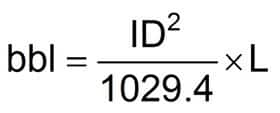Note  Drilling / Mud Engineer shall use inside diameter (ID) for casing and bit diameter for open-hole calculations. Do not allow for open-hole washout unless values are known from fluid (mud) logging data or caliper logs. Annular or casing capacity tables can be used in lieu of the formulae.

### Pipe Capacity

Equation 14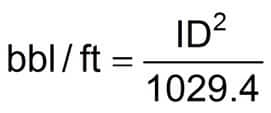or Equation 15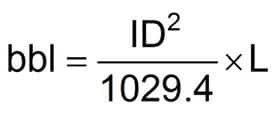Where:

ID = Inside diameter of drillpipe. (in.)

Use equivalent IDs from pipe tables in this section.

L = Length (ft)

### Pipe Displacement Obtained from Pipe Tables

Because of the varying thicknesses of the various types of tool joints, read displacements from pipe tables rather than calculate them, unless tool joint specifications are available.

#### Annular Volume

Equation 16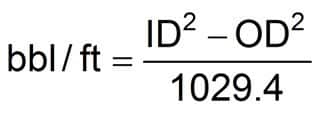or

Equation 17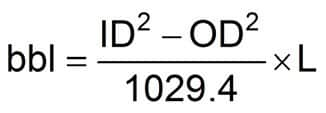Where:

• ID = Inside diameter of casing or bit diameter (in.)
• OD = Outside diameter of drillpipe or drill collars (in.)
• L = Annular section length (ft)

#### Annular Velocity

Equation 18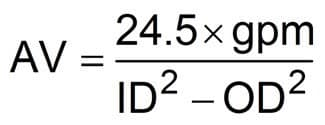Where:

• AV = Annular velocity, (ft/min)
• gpm = gal/min
• ID = Inside diameter of the hole or casing (in.)
• OD = Outside diameter of the pipe or collars (in.)

#### Bottoms Up (Strokes or Time)

Equation 19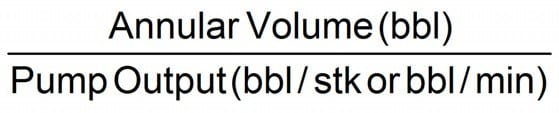#### Total Circulating Displacement (Strokes or Time)

Equation 20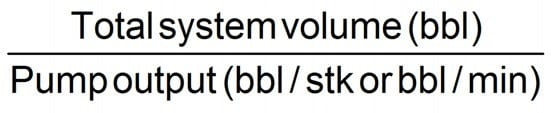Where:

• Total system volume = Surface volume + Pipe capacity + Annular volume

#### Hole Volume Displacement (Strokes or Time)

Equation 21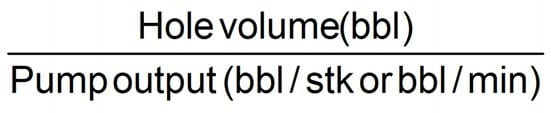Where:

• Hole volume = Annular volume + Drillpipe capacity
• Hole volume = Open hole capacity – Drillstring displacement

#### Drilling Mud Circulating Time Calculations

##### Nomenclature
• Annulus capacity — Anncap
• Pump output — Q
• Drill string capacity — DScap

Equation 22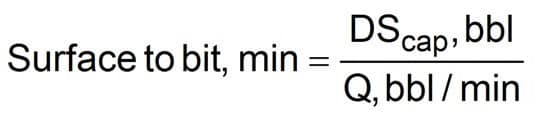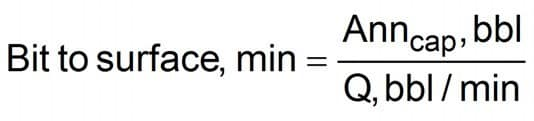Equation 23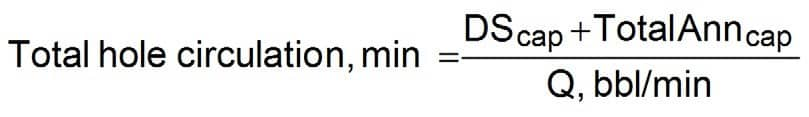Equation 24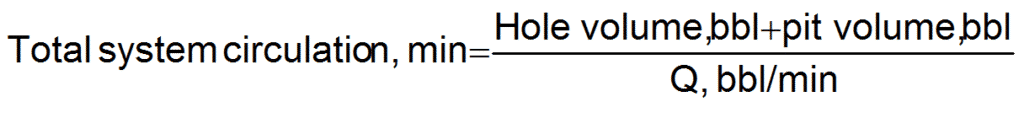### Density and Volume Calculations

#### Density Increase Using (Barite)

Equation 25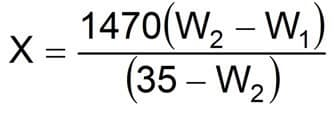Where:

• X = Sacks of BAR/100 bbl fluid (100 lbm sacks)
• W1 = Initial fluid density (lbm/gal)
• W2 = Desired fluid density (lbm/gal)

If using Barite 4, replace 1470 with 1,435 and 35 with 34 in the above equation.

#### Volume Increase Using (Barite)

Equation 26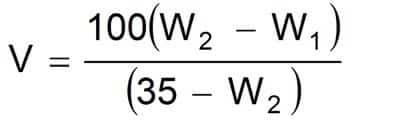Where:

• V = Volume increase/100 bbl fluid
• W1 = Initial fluid density (lbm/gal)
• W2 = Desired fluid density (lbm/gal)

If using Barite 4, replace 35 with 34 in the above equation.

#### Density Increase Using (Hematite)

Equation 27Where:

• X = Sacks of DENSIMIX/100 bbl fluid (100 lbm sacks)
• W1 = Initial fluid density (lbm/gal)
• W2 = Desired fluid density (lbm/gal)

#### Volume Increase Using (Hematite)

Equation 28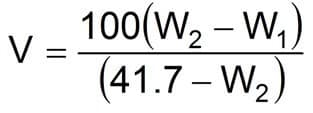Where:

• V = Volume increase/100 bbl of fluid
• W1 = Initial fluid density (lbm/gal)
• W2 = Desired fluid density (lbm/gal)

#### Density Increase Using (CaCO3)

Equation 29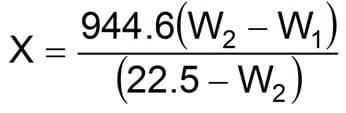Where:

• X = Sacks of MIL-CARB/100 bbl fluid (100 lbm sacks)
• W1 = Initial fluid density (lbm/gal)
• W2 = Desired fluid density (lbm/gal)

#### Volume Increase Using (CaCO3)

Equation 30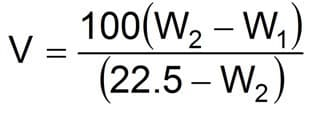Where:

• V = Volume increase/100 bbl of fluid
• W1 = Initial fluid density (lbm/gal)
• W2 = Desired fluid density (lbm/gal)

#### Weight Reduction with Freshwater

Equation 31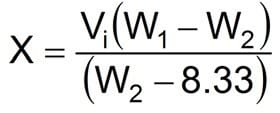Where:

• X= bbl of water required
• Vi= Initial volume (bbl)
• W1 = Initial fluid density (lbm/gal)
• W2 = Desired fluid density (lbm/gal)

#### Weight Reduction with Oil

Equation 32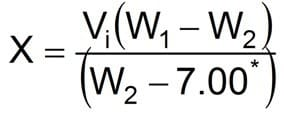The specific gravity of oil = 0.84

Where:

• X = bbl of oil required
• Vi = Initial volume (bbl)
• W1 = Initial fluid density (lbm/gal)
• W2 = Desired fluid density (lbm/gal)

#### Mixing Liquids of Different Densities

Equation 33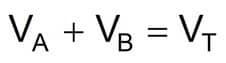Equation 34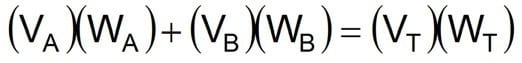Where:

• VA = Volume of first fluid (bbl)
• VB = Volume of second fluid (bbl)
• VT = Final volume (bbl)
• WA = Density of first fluid (lbm/gal)
• WB = Density of second fluid (lbm/gal)
• WT = Density of combined fluids or final weight (lbm/gal)

Note  Drilling / Mud Engineer shall know that this drilling fluid calculations formula assumes fluids are totally miscible, no precipitation reactions occur, and fluids are of comparable salinity. This equation does not apply to the mixing of high-density brine fluids.

#### Fluid Density Increase for Slugging Pipe

Equation 35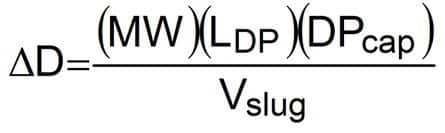Where:

• ∆D = Fluid density increase (lb/gal)
• MW = Current fluid density (lb/gal)
• DPcap = Drillpipe capacity (bbl/ft)
• Vslug = Slug volume, usually 30 to 50 bbl
• LDP = Desired length of dry pipe, usually 500 to 800 ft

### Vertical Depth vs. Deviated Hole Calculations

#### True vertical depth (average angle method)

Equation 36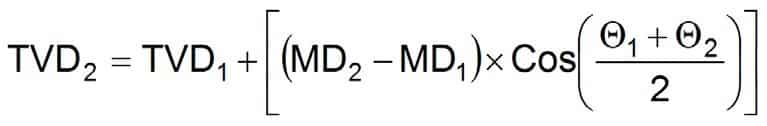#### Horizontal displacement (average angle method)

Equation 37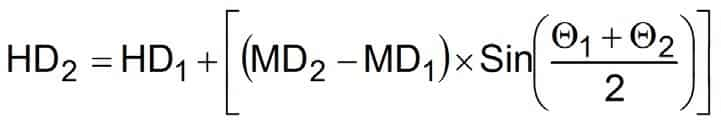#### Kick-off point determination

Equation 38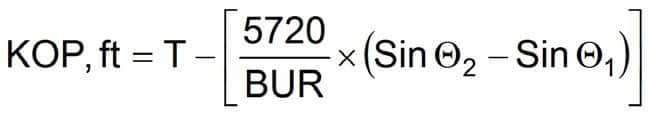Where (all depths in feet):

• TVD1 = True vertical depth at point 1
• TVD2 = True vertical depth at point 2
• MD1 = Measured depth at point 1
• MD2 = Measured depth at point 2
• HD1 = Horizontal displacement at point 1
• HD2 = Horizontal displacement at point 2• θ1 = Deviation at point 1
•θ2 = Deviation at point 2
• T = Target depth
• BUR = Build-up rate (°/100 ft)

Note  This method of drilling fluid calculations assumes that the course length between point 1 and point 2 is a straight line. The direction and inclination are also assumed to be the averages of the values measured at each point. The method will be less accurate when applied over long course lengths, but it is a good approximation for most drilling / mud engineering calculations.

Drilling mud / fluid Calculations (mud engineer calculations) for deviated wells must use measured depth when calculating volumes and vertical depth when calculating equivalent fluid weights.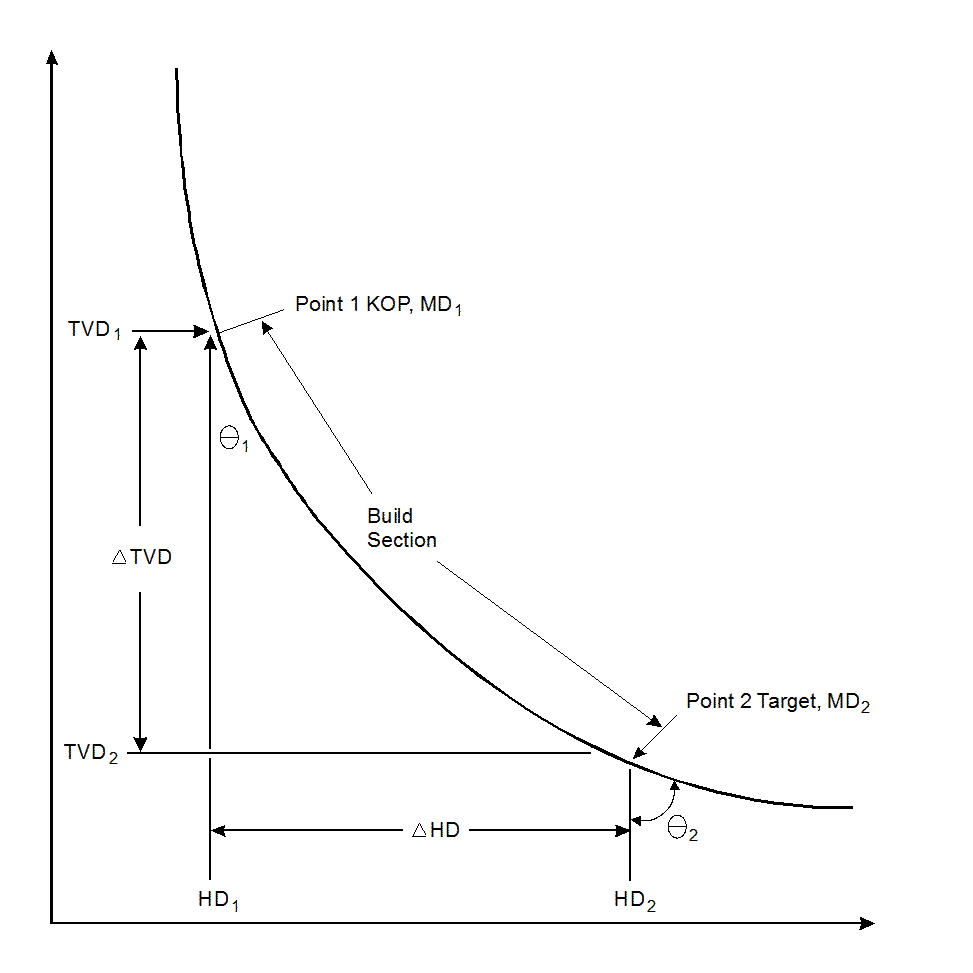Figure 2: Well Profile for drilling fluids calculations Using Average Angle Method

### BuoyancyFigure 3: Buoyancy GraphIn this article we introduce a simple guide for drilling fluid calculations for mud engineering, preparations, system, volumes and more.

## Drilling Fluids Calculations For Drilling & Mud Engineer

Our Facebook Page Pool Remodeling, Pool Repairs, Pool Company

Call US:281-224-6739

Email us at: [email protected]